Click Question to Get Free Answers >

# Herons Formula NCERT Solutions : Class 9 Maths

## NCERT Solutions for Class 9 Maths : Herons FormulaFilter
Filters :
Classes
•
•
•
•
•
•
•
Chapters
•
•
•
•
•
•
•
•
•
•
• 7 More
Exercises
•
•
•

### NCERT Class 9 | HERONS FORMULA | Exercise 02 | Question No. 07

A kite in the shape of a square with a diagonal 32 cm and an isosceles triangle of base 8 cm and sides 6 cm each is to be made of three different shades as shown in Fig. 12.17. How much paper of each shade has been used in it?### NCERT Class 9 | HERONS FORMULA | Exercise 02 | Question No. 08

A floral design on a floor is made up of 16 tiles which are triangular, the sides of the triangle being 9 cm, 28 cm and 35 cm (see Fig. 12.18). Find the cost of polishing the tiles at the rate of 50 p"\ "p e r"\ "c m^2 .### NCERT Class 9 | HERONS FORMULA | Exercise 01 | Question No. 01

A traffic signal board, indicating ‘SCHOOL AHEAD’, is an equilateral triangle with side ‘a’. Find the area of the signal board, using Heron’s formula. If its perimeter is 180 cm, what will be the area of the signal board?### NCERT Class 9 | HERONS FORMULA | Exercise 02 | Question No. 05

A rhombus shaped field has green grass for 18 cows to graze. If each side of the rhombus is 30 m and its longer diagonal is 48 m, how much area of grass field will each cow be getting?Doubtnut one of the best online education platform provides free NCERT Solutions of Maths for Class 9 Herons Formula which are solved by our maths experts as per the NCERT (CBSE) guidelines. We provide the solutions as video solutions in which concepts are also explained along with answer which will help students while learning , preparing for exams and in doing homework. These Solutions will help to revise complete syllabus and score more marks in examinations.

Get here free, accurate and comprehensive NCERT Solutions for Class 9 Maths Herons Formula which have been reviewed by our maths counsellors as per the latest edition following up the CBSE guidelines. We provide video solutions in which solutions to all the questions of NCERT Class 9 Maths textbook are explained in step by step and detailed way.

## The Topics of the Chapter Herons Formula are :

HERONS FORMULA

INTRODUCTION

It contains these exercises along with solved examples. All the exercises are solved in the video. Select the exercise to view the solutions exercisewise of the Chapter Herons Formula:

## NCERT Solutions Class 9 Maths Chapter Herons Formula Exercises:

We have covered all the exercises and also Solved examples in the videos. Along with the practise exercise Students should also practise solved examples to clear the concepts of the Herons Formula. If incase you have any doubt you can watch the solutions for the given questions.Watch the solutions for the given questions in which it is also explained in the video steps to solve the questions along with answers.

# NCERT Class 9 Solutions Chapter 12 Heron’s Formula

CBSE board is one of the renowned names in the Indian education system under which lakhs of students pursue their elementary education. Where NCERT books are a compulsory partner of students who are appearing for CBSE board exams, some students find it better to learn concepts with NCERT help guides. The reason behind almost every student have an NCERT book with them is because NCERT syllabus books are enough to excel in CBSE exams.

The NCERT books are thoroughly known for their easy language that covers tricky formulas, effective tips, and add-on exercises help students further to practice more and indulge more. For students of class 9, this book is a boon as it is the initial stage for the preparation of class 10 board examinations. Be it any competitive entrance exam, math is one subject that stays tight. The NCERT books contain extra formulas, time-efficient tips, and solved questions that altogether help you to practice better for exams. Math is indeed a subject that requires timely revisions and extra efforts; if a student routinely studies through NCERT and solves most of the question it has, then he/she definitely can clear the competitive exams.

At Doubtnut, an online tutorial platform, we provide all the solutions for the NCERT textbook exercises. NCERT solution for Class 9 maths Chapter 12 Heron’s formula is presented in the form of videos that can be accessed from anywhere and at any time. The students can rely on these solution videos as all problems, including the complex ones are broken down into simpler solutions by our experts who are available to solve students queries whenever they require. Theories, as well as exercises of the chapter, are available on our website.  Moreover, the Doubtnut app makes it easier and convenient for the students to pass the examination with flying colors.

Introduction – Heron’ formula Class 9 Maths NCERT Solutions

In previous chapters, students have learned about figures of different sizes and shapes like triangle, square, rectangle and quadrilateral. Students have learned to take out areas and of these figures.

If we take an example of our room as much distance, we walk around is said to be the parameter and the size of the floor of the room is considered as its area.

Therefore, we know the measurement unit of length and breadth is described as meter or centimetre.

For the measurement of a unit of area of a plane figure is taken as m2 (square meter) and cm2 (centimetre square)

So the area of the triangle is equal to ½ x base x height.

Now further in this chapter students will be learning about the new formula to find out areas of different triangles with the help of Heron's formula. Now let us recall and study the chapter in detail now.

## Topics and Subtopics of NCERT Class 9 Maths Chapter 12 – Heron’s Formula

Chapter Heron’s Formula is further divided into topics and subtopics in which the content of previous knowledge of the student is given as well as the current syllabus is covered in the form of theory and exercises. Knowing the topics and subtopics of a given chapter helps the learners be aware of the whole content in a short form. The knowledge of topics and subtopics help students to plan their studies accordingly in an organized manner. Let us look at the topics and Subtopics of NCERT Class 9 Maths Chapter 12 – Heron’s Formula, according to which the solutions are worked out on the website:

### Who was Heron?

Heron was a well-known encyclopaedic writer in the field of Math and physics. He has written an ample number of books on mathematical topics. He has written three books on problems of mensuration.

Out of which Book I deal with areas of triangle, square, rectangle, cylinder, cone and other various special figures. According to his book, the formula for the area of the triangle is derived on the basis of three sides of the triangle. It means if we don’t know the height of a triangle heron's formula can be used for taking out the area of a triangle with the help of three sides of the triangle.

### What is Heron’s formula

Heron gave the formula to find out the area of a triangle which is also called as hero's formula. This formula is helpful when the height of the triangle is not known or it is not easy to find the height by any method. The formula is given as:-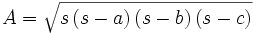In this formula a, b, c are the sides of the triangle and s is the semi perimeter or we can say half perimeter of a triangle.

S can be written as shown below on the basis of above formula

{\displaystyle A={\sqrt {s(s-a)(s-b)(s-c)}},} s =   a+b+c

2

Application of Heron's formula for different triangles

Examples – We can see a triangle with three sides as 16, 22 and 12m. Let's see how we can find the area of the given triangle through heron’s formula -

{\displaystyle s={\frac {a+b+c}{2}}.}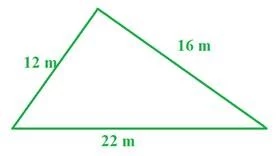In the first step lets find it half perimeter which is as –

Perimeter of triangle is equal to 12 + 16 + 22 = 50

Therefore, the half perimeter is equal to half of the value of s

Hence 50/2 = 25

i.e. s = 25

Now substituting the value of s in heron’s formula we get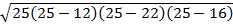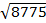A = 93.7 m2

Let’s take a right-angle triangle with sides given as 6m, 8m, and 10m

Let’s find out half perimeter of right-angle triangle

Perimeter of triangle is equal to 10 + 6 + 8 = 24

Therefore, the half perimeter is equal to half of the value of s

Hence 24/2 = 12

i.e. s = 12

A =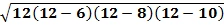A =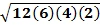A = √576

Therefore, the area of the right-angle triangle is equal to 24m2

### Application of Heron's formula in quadrilateral

We can apply Heron's formula to find out the area of the quadrilateral as follows

For using this formula, a quadrilateral is divided into two triangular parts then we find out the area of both the triangles and will add both to get the area of a quadrilateral.

Example -

Kamla has a triangular field with sides 240 m, 200 m, 360 m, where she used to

grow wheat. In another triangular field with sides 240 m, 320 m, 400m adjacent to the

previous field, she wanted to grow potatoes and onions. She divided the field into two parts by joining the mid-point of the longest side to the opposite vertex and grew potatoes in one part and onions in the other part. How much area (in hectares) has been used for wheat, potatoes and onions?

(1 hectare = 10000 m2)

Let us consider ABC as the field where wheat is grown. Also let ACD be the field

which has been divided in two parts by joining C to the mid-point E of AD. For the

area of triangle ABC, we have

a = 200 m, b = 240 m, c = 360 m

Therefore, s = 200 + 240 + 360

2

m = 400 m.

So, area for growing wheat

=  √400(400 â”€ 200) (400 – 240) (400 – 360)m2

=    √400 x 200 x 160 x 40m2

=   16000 √ 2 m2 = 1.6 × √2 hectares

= 2.26 hectares (nearly)

We will calculate the area of triangle ACD now.

Here, we have s = 240 +320+400 m

2

Therefore s= 480 m.

So, area of triangle ACD =  √480(480 â”€ 240) (480 â”€ 320) (480 â”€ 400) m2

= √480x240x160x80 m2

= 38400m2 = 3.84 hectares

Therefore, area for growing potatoes = area for growing onions

= (3.84 ÷2) hectares = 1.92 hectares.

Hence, through applying heron’s formula in above example we have found area of the quadrilateral.

Significance of NCERT Book Class 9

1. The NCERT Mathematics book for Class 9 provides explanations of each topic that help students to brush up their concepts right from the base.
2. Most of the students refer to these help guides to unleash the route to success as it allow them to indulge basic concepts rather than solving the formula-based questions directly.
3. We know that maths is not literature that can be learned through reading. Instead, it is a subject requires timely learning of formulas and practicing the questions as much as a student calibre allows.
4. The NCERT mathematics book for Class 9 have multiple practice questions & exercises to solve.

The students are benefitted while practicing from the NCERT book and if they have any doubts it can be cleared with the help of online tutorials videos which are given on doubtnut.com, where the subject matter experts are available to help the students. On our website, all of these concepts are taught in video format and this helps the students to learn the lessons with better concentration. The lessons taught in a step-by-step manner by an expert teacher, helps in understanding the concepts in a better way. All the doubts are cleared by our experts in an easy and efficient manner. Thus, Doubtnut is the right platform and students should download this app for easy access to their queries and getting success. Our website makes the perfect tutorial platform for the students to face their exams in a stress-free way!